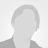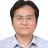# An algorithm of calculating transport parameters of thermoelectric materials using single band model with optimized integration methods

Published: 30 Oct 2019 | Version 1 | DOI: 10.17632/8r5ybpjrbb.1
Contributor(s):
• Kuo-Chuan Chang,Kuo-Chuan Chang
National Changhua University of Education
• Chia-Jyi LiuMaterials Science
National Changhua University of Education

## Description of this data

We develop an algorithm called SPBcal under Java SE runtime environment 1.8.0_131 to calculate transport parameters of thermoelectric materials within the framework of single parabolic band (SPB) model. For calculating Fermi-Dirac integrals, SPBcal is implemented by a double exponential transformation combined with trapezoidal rule to calculate F_1(n) and F_2(n) and the trapezoidal rule with pole correction to calculate F_{-1/2}(n) and F_{1/2}(n), with which good accuracy of the transport parameters as a function of temperature can be obtained with very low execution time of the algorithm as long as the experimentally determined Seebeck coefficients are given. The SPBcal is tested and the results are consistent with the data reported in the literature.

## Experiment data files

This data is associated with the following publication:

An algorithm of calculating transport parameters of thermoelectric materials using single band model with optimized integration methods

• ### Version 1

2019-10-30

Published: 2019-10-30

DOI: 10.17632/8r5ybpjrbb.1

### Cite this dataset

Chang, Kuo-Chuan; Liu, Chia-Jyi (2019), “An algorithm of calculating transport parameters of thermoelectric materials using single band model with optimized integration methods”, Mendeley Data, v1 http://dx.doi.org/10.17632/8r5ybpjrbb.1

## Statistics

Views: 13

### Categories

Computational Physics, Thermoelectrics